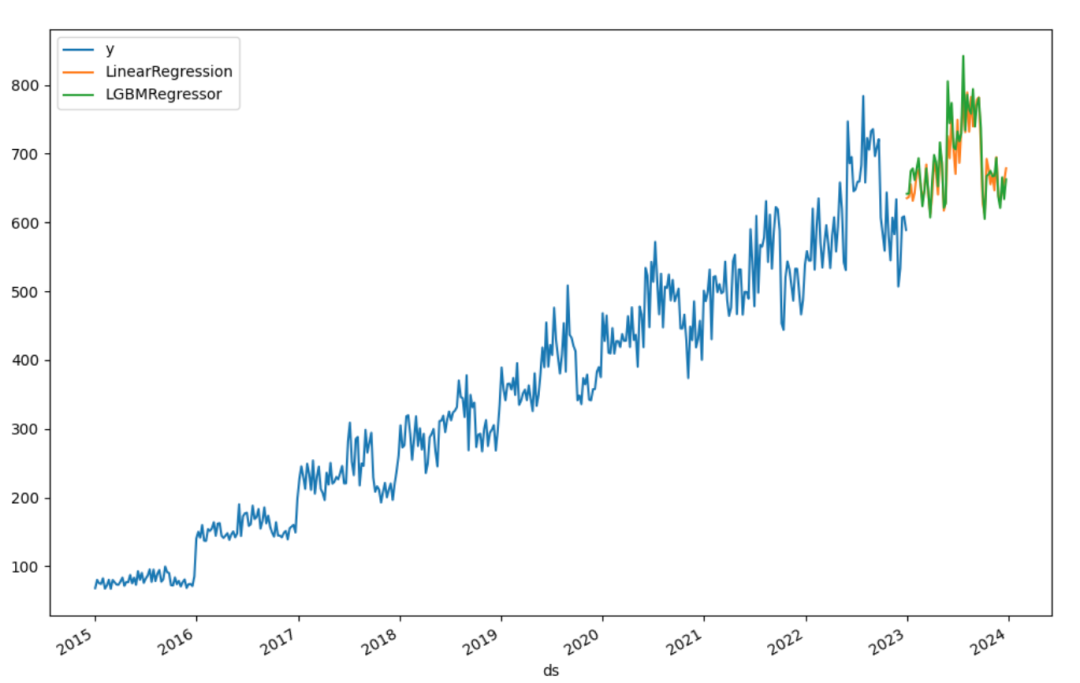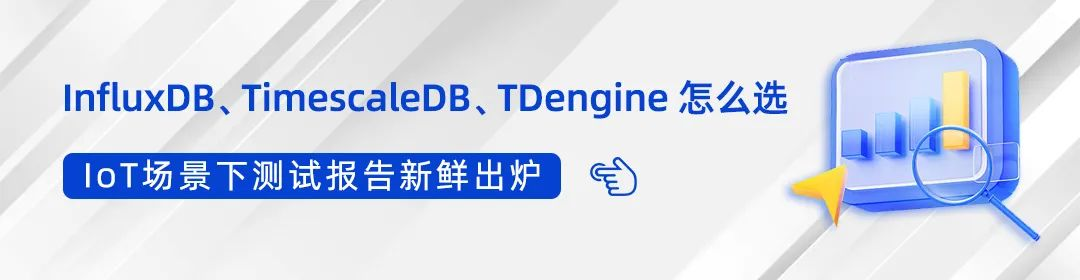# 如何用 TDengine 预测 “未来”？

06/27 17:34

TDengine™ 是一种开源的云原生时序数据库（Time Series Database，TSDB），专为物联网（IoT）、连接汽车和工业物联网进行了优化。它能够高效地实时摄取、处理和监控一天内由数十亿个传感器和数据收集器产生的PB级别的数据。

git clone https://github.com/sangshuduo/td-forecasting

# if you are using Ubuntu 20.04 Linuxsudo apt install python3-pyqt5# 如果 PyQT5 运行失败，可能需要安装sudo apt-get install libxcb-xinerama0python3 -m pip install -r requirements.txt

python3 mockdata.py

python3 forecast.pymockdata.py

...def insert_rec_per_month(conn, db_name, table_name, year, month):    increment = (year - 2014) * 1.1    base = int(10 * increment)    if month < 10 and month > 5:        factor = 10    else:        factor = 8    for day in range(1, monthrange(year, month) + 1):        num = base * randint(5, factor) + randint(0, factor)        sql = f"INSERT INTO {db_name}.{table_name} VALUES ('{year}-{month}-{day} 00:00:00.000', {num})"        try:            conn.execute(sql)        except Exception as e:            print(f"command: {sql}")            print(e) ...

forecast.py

import argparseimport lightgbm as lgbimport matplotlib.pyplot as pltimport mlforecastimport pandas as pdfrom mlforecast.target_transforms import Differencesfrom sklearn.linear_model import LinearRegressionfrom sqlalchemy import create_engine, text...

• Lightgbm 是一个 Python 模块，支持 LightGBM 算法，它是一个使用基于树的学习算法的梯度提升框架。
• Matplotlib 是最流行的 Python 模块之一，用于可视化。
• Mlforecast 是一个框架，用于使用机器学习模型执行时间序列预测。
• Pandas 是支持数据操作的最流行的模块。
• Sklearn 是一个支持流行的数据科学/机器学习算法的模块。
• SQLAlchemy 是 Python SQL 工具包和对象关系映射器，它为应用程序开发人员提供了完整的 SQL 功能和灵活性。

...    engine = create_engine("taos://root:taosdata@localhost:6030/power")    conn = engine.connect()    print("Connected to the TDengine ...")    df = pd.read_sql(        text("select _wstart as ds, avg(num) as y from power.meters interval(1w)"), conn    )    conn.close()...

TDengine Python 连接器提供符合 Python 数据库 API 规范 v2.0（PEP 249）的接口。DBAPI 是“Python 数据库 API 规范”的缩写。这是 Python 中广泛使用的规范，用于定义所有数据库连接包的常用用法模式。DBAPI 是一个“低级别”API，通常是 Python 应用程序中用于与数据库交互的最低级别系统。SQLAlchemy 的方言系统是围绕DBAPI 的操作构建的，提供适用于特定数据库引擎的特定 DBAPI 的各个方言类。

...    df.insert(0, column="unique_id", value="unique_id")    print("Forecasting ...")    forecast = mlforecast.MLForecast(        models=[LinearRegression(), lgb.LGBMRegressor()],        freq="W",        lags=,        target_transforms=[Differences()],    )    forecast.fit(df)    predicts = forecast.predict(52)    pd.concat([df, predicts]).set_index("ds").plot(figsize=(12, 8)) ...

Show up or dump to file

...    if args.dump:        plt.savefig(args.dump)    else:        plt.show()

Python 代码提供了一个参数"--dump"，让用户可以决定将结果转储到图片中进行后续处理，或者立即在屏幕上显示结果。

往期推荐👇  点击阅读原文，快速体验 TDengine 3.0 ！0 评论
0 收藏
0